• ## 概率图模型的简介

千次阅读 2020-03-13 00:19:49
概率图模型的表示框架下，系统的联合概率分布表示为局部变量势函数的连乘积，该表示框架不仅避免了对复杂系统的联合概率分布直接进行建模，而且易于在图模型建模中引入先验知识。   2012年图灵奖颁给UCLA的Judea...
什么是概率图模型
概率图模型是概率论与图论相结合的产物，为统计推理和学习提供了一个统一的灵活框架。概率图模型提供了一个描述框架，使我们能够将不同领域的知识抽象为概率模型，将各种应用中的问题都归结为计算概率模型里某些变量的概率分布，从而将知识表示和推理分离开来。
利用图模型进行实际问题求解的时候包括两步：

知识表示，概率图模型的表示建模；
将实际问题转化为一个推理的问题。

这样就把知识表示和推理分离开来了。上图中的左图是一个图，图中含有节点，有边，每一个节点表示我们所研究问题的一个变量，变量之间的边表示：变量局部的依赖关系。右边所表示的是系统的联合概率分布，这个问题包含$N$维变量，每个变量包含$k$个可能的取值。概率图模型就是在这样一个图上定义一个联合概率分布，建模完成以后再在这个概率图模型上进行推理计算。从而解决一些实际的应用问题。
概率图模型用节点表示变量，节点之间的边表示局部变量间的概率依赖关系。在概率图模型的表示框架下，系统的联合概率分布表示为局部变量势函数的连乘积，该表示框架不仅避免了对复杂系统的联合概率分布直接进行建模，而且易于在图模型建模中引入先验知识。
2012年图灵奖颁给UCLA的Judea Pearl教授，奖励其在贝叶斯网络上的开创性工作，贝叶斯网络属于有向的概率图模型。消息传递是概率图模型中消息传递的一种比较创新的机制。通过消息传递可以把全局的概率推理转化为图上的局部变量之间的消息传递。能够大大降低推理的复杂度。概率图模型统一了目前广泛应用的许多统计模型和方法。比如：

马尔可夫随机场 (MRF)一种无向的概率图模型，在图像处理，统计物理学上有非常广泛的应用。
条件随机场(CRF)，可以应用于NLP(自然语言处理)
隐马尔可夫模型(HMM)
多元高斯模型
Kalman滤波、粒子滤波、变分推理

这些不同的统计模型和方法都可以纳入到概率图模型这个统一的框架里面。也就是说上述的方法是特殊的概率图模型，都可以归纳到概率图模型的大类里面。
概率图模型举例：
贝叶斯网贝叶斯网络(Bayesian network)又称信度网络(belief network)，是Bayes方法的扩展，是目前不确定知识表达和推理领域最有效的理论模型之一。
如上图所示，每一个节点表示一个变量，变量可以是任何问题的抽象，如：测试值，观测现象，意见征询等。变量之间的边用有向的箭头连接,贝叶斯网络是一个有向无环图(Directed Acyclic Graph,DAG)，所以贝叶斯网络是一种有向的概率图模型。贝叶斯网络中可以定义一个联合概率分布，具体表示为每个节点的条件概率连乘积的形式：
$p(\mathbf{x}) = \prod_{s}p_{s}(x_{s}|x_{\pi{(s)}})$
适用于表达和分析不确定性和概率性的事件，应用于有条件地依赖多种控制因素的决策，可以从不完全、不精确或不确定的知识或信息中做出推理。
机器学习里面有两派，一派是频率派、一派是贝叶斯派。在频率派中参数是多少就是多少，而在贝叶斯派中觉得所有的数值都是随机变量，按照某一个概率出现。图模型、无监督算法更多地关心的是参数的估计，也就是贝叶斯派的思想。
在数据科学中的核心问题就是利用已知数据建立一个模型或者说是一种分布，然后去预测一些东西。那现在的问题就是如何去建立这样一个模型。数据建模过程中的难点在于如何在数据维度分布很高的情况下获取数据之间模型的关系。
比如说你想要去建模一个明天什么天气下穿什么衣服的模型$p(t,c)=p(t)p(c|t)$，它会等于明天温度为冷热的概率$p(t)$和在这个温度下，穿什么衣服的条件概率$p(c|t)$的乘积。
我们有两种办法来做一种是有向(directed graphs)的贝叶斯网络(Bayesian network)，另一种是无向(undirected graphs)的马尔可夫网络(Markov network)。

贝叶斯网络：

$p(t,c)=p(t)p(c|t)$

马尔可夫网络：

$p(t,c)=\frac{e^{\phi(t,c)}}{\sum_{t^{\prime},c^{\prime}}e^{\phi(t^{\prime},c^{\prime})}}$
Bayesian Network
一个简单的贝叶斯网络可表示为如下形式：其联合概率分布可表示为：
$p(a,b,c)=p(c|a,b)p(b|a)p(a)$
对于稍微复杂一点的网络，其结构可表示为：如果直接建模的话，七个变量直接乘在一起，建模空间比较大，而使用贝叶斯网络的话，数据的联合概率分布可表示为：
$\begin{array}{l} p\left(x_{1}, x_{2}, x_{3}, x_{4}, x_{5}, x_{6}, x_{7}\right)= \\ p\left(x_{1}\right) p\left(x_{2}\right) p\left(x_{3}\right) \\ p\left(x_{4} | x_{1}, x_{2}, x_{3}\right) p\left(x_{5} | x_{1}, x_{3}\right) \\ p\left(x_{6} | x_{4}\right) p\left(x_{7} | x_{4}, x_{5}\right) \end{array}$
建模空间缩小了。其实上述公式也非常好理解，你只需要注意箭头指向的方向，就会很容易发现其中的规律。
举例
假设我们现在有一些训练数据$D=\{(x_{i},t_{i})\}$，$x$是data，t是label。假设建立一个带有参数$\mathbf{w}$的模型，这个参数服从某个高斯分布。有：
\begin{aligned} p(\mathbf{w} | \alpha) &=\mathcal{N}(\mathbf{w} | \mathbf{0}, \alpha) \\ p\left(t_{i} | x_{i}, \mathbf{w}, \sigma^{2}\right) &=\mathcal{N}\left(t_{i} | \mathbf{w}^{\top} x_{i}, \sigma^{2}\right) \\ p\left(\mathbf{t}, \mathbf{w} | \mathbf{x}, \alpha, \sigma^{2}\right) &=p(\mathbf{w} | \alpha) \prod_{i=1}^{N} p\left(t_{i} | x_{i}, \mathbf{w}, \sigma^{2}\right) \end{aligned}
给定超参数$\alpha$，可以得到$\mathbf{w}$，得到$\mathbf{w}$之后，对于任意一个$x_{i}$我都能得到一个label $t_{i}$的概率，也就是可以通过Gaussian可以采出来一个$t_{i}$，并且这个高斯的分布也是不确定的。也可以表示为：
$p(\mathbf{t}, \mathbf{w})=p(\mathbf{w}) \prod_{i=1}^{N} p\left(t_{i} | \mathbf{w}\right)$
用图形化的语言可表示为如下形式：由于$t_{1}$到$t_{N}$其实是一类性质。图形化语言可简画为如下形式：预测的标签是一组随机变量，依据真实标签(数据)，likelihood后验概率分布可以得到。其后验概率(Posterior Distribution) $p(\mathbf{w|t})$ 可表示为：
$p(\mathbf{w} | \mathbf{t})=\frac{p(\mathbf{w}) p(\mathbf{t} | \mathbf{w})}{p(\mathbf{t})}$
由于$p(t)$是真实标签，其概率不变，上式正比于：
$p(\mathbf{w}) \prod_{i=1}^{N} p\left(t_{i} | \mathbf{w}\right)$
也就是等于先验分布$p(\mathbf{w})$与Data likelihood的乘积。由此问题转变为最大化后验概率分布估计的问题：
$\max _{\mathbf{w}} p(\mathbf{w} | \mathbf{t})=\max _{\mathbf{w}} p(\mathbf{w}, \mathbf{t})=\max _{\mathbf{w}} p(\mathbf{w}) p(\mathbf{t} | \mathbf{w})$
将其展开，代入高斯函数，可以得到：
\begin{aligned} p(\mathbf{w}) p(\mathbf{t} | \mathbf{w}) &=p(\mathbf{w} | \alpha) \prod_{i=1}^{N} p\left(t_{i} | x_{i}, \mathbf{w}, \sigma^{2}\right) \\ &=\mathcal{N}(\mathbf{w} | \mathbf{0}, \alpha) \prod_{i=1}^{N} \mathcal{N}\left(t_{i} | \mathbf{w}^{\top} \mathbf{x}, \sigma^{2}\right) \\ &=\frac{1}{\sqrt{(2 \pi \alpha)^{d}}} \exp \left(-\frac{\mathbf{w}^{\top} \mathbf{w}}{2 \alpha^{d}}\right) \prod_{i=1}^{N} \frac{1}{\sqrt{2 \pi \sigma^{2}}} \exp \left(-\frac{\left(t_{i}-\mathbf{w}^{\top} \mathbf{x}\right)^{2}}{2 \sigma^{2}}\right) \end{aligned}
对其取log，得到：
$\log p(\mathbf{w}) p(\mathbf{t} | \mathbf{w})=-\frac{\mathbf{w}^{\top} \mathbf{w}}{2 \alpha^{d}}-\sum_{i=1}^{N} \frac{\left(t_{i}-\mathbf{w}^{\top} \mathbf{x}\right)^{2}}{2 \sigma^{2}}+\text { const }$
等价于：
$\min _{\mathbf{w}} \frac{\sigma^{2}}{\alpha^{d}}\|\mathbf{w}\|^{2}+\sum_{i=1}^{N}\left(t_{i}-\mathbf{w}^{\top} \mathbf{x}\right)^{2}$
它与带有均方误差的回归问题是等价的。上述过程是模型的求解过程，对于新的数据，如何做推断呢？给定先验和数据的likelihood，可以得到$\mathbf{w}$，之后再得到新的数据的预测标签。
$p\left(\hat{t}, \mathbf{t}, \mathbf{w} | \hat{x}, \mathbf{x}, \alpha, \sigma^{2}\right)=\left[\prod_{i=1}^{N} p\left(t_{i} | x_{i}, \mathbf{w}, \sigma^{2}\right)\right] p(\mathbf{w} | \alpha) p\left(\hat{t} | \hat{x}, \mathbf{w}, \sigma^{2}\right)$
Marginalize out the coefficients  $\mathbf{w}$(也就是最开始不考虑 $\mathbf{w}$)：
\begin{aligned} p\left(\hat{t} | \hat{x}, \mathbf{x}, \mathbf{t}, \alpha, \sigma^{2}\right) &=\frac{p\left(\hat{t}, \mathbf{t} | \hat{x}, \mathbf{x}, \alpha, \sigma^{2}\right)}{p(\mathbf{t})} \\ & \propto p\left(\hat{t}, \mathbf{t} | \hat{x}, \mathbf{x}, \alpha, \sigma^{2}\right) \\ &=\int p\left(\hat{t}, \mathbf{t}, \mathbf{w} | \hat{x}, \mathbf{x}, \alpha, \sigma^{2}\right) d \mathbf{w} \end{aligned}马尔可夫随机场
马尔可夫随机场(Markov Random Field)马尔可夫随机场是一种无向的概率图模型，这个无向的概率图模型上的联合概率分布可以表示成节点势函数和边上的势函数连乘积的形式。
$p(\mathbf{x})=\frac{1}{Z} \prod_{p} \phi_{p}\left(x_{p}\right) \prod_{(p, q)} \phi_{p q}\left(x_{p}, x_{q}\right)$
马尔可夫随机场是建立在马尔可夫模型和贝叶斯理论基础之上的，它包含两层意思：一是什么是马尔可夫，二是什么是随机场。
马尔可夫性质：它指的是一个随机变量序列按时间先后关系依次排开的时候，第N+1时刻的分布特性，与N时刻以前的随机变量的取值无关。拿天气来打个比方。如果我们假定天气是马尔可夫的，其意思就是我们假设今天的天气仅仅与昨天的天气存在概率上的关联，而与前天及前天以前的天气没有关系。其它如传染病和谣言的传播规律，就是马尔可夫的。
随机场：当给每一个位置中按照某种分布随机赋予相空间的一个值之后，其全体就叫做随机场。我们不妨拿种地来打个比方。其中有两个概念：位置（site），相空间（phase space）。“位置”好比是一亩亩农田；“相空间”好比是种的各种庄稼。我们可以给不同的地种上不同的庄稼，这就好比给随机场的每个“位置”，赋予相空间里不同的值。所以，俗气点说，随机场就是在哪块地里种什么庄稼的事情。
马尔可夫随机场：马尔科夫随机场是具有马尔科夫特性的随机拿种地打比方，如果任何一块地里种的庄稼的种类仅仅与它邻近的地里种的庄稼的种类有关，与其它地方的庄稼的种类无关，那么这些地里种的庄稼的集合，就是一个马尔可夫随机场。
马尔可夫网络(Markov Networks)与贝叶斯网络的区别就在于，它是无向图，他没有箭头。在这种情况下任何两个变量之间存在连边,也存在独立性。 这种连接并没有因果关系，并没有B导致C或C导致B这样一种假设。这样一种假设相比于贝叶斯网络更容易去定义两个变量之间的独立性。  如上图所示,A和B两个节点之间的路径都被C包含，那么A，B这两个节点会条件独立于C这个节点。也就是说给定C节点，A和B这两个节点条件独立。
$A \perp \!\!\! \perp B | C$
由于上述性质，它相对于贝叶斯网络会简单一点。马尔可夫网络的blanket就是他的neighboring nodes，而贝叶斯网络还包括其儿子节点的其他父亲节点，去影响其分布。在这种情况下用数学形式表达条件独立的话就比较简单了，Consider two nodes $x_{i}$ and $x_{j}$ that are not connected by a link, then these variables must be conditionally independent given all other nodes in the graph：
$p\left(x_{i}, x_{j} | \mathbf{x}_{\backslash\{i, j\}}\right)=p\left(x_{i} | \mathbf{x}_{\backslash\{i, j\}}\right) p\left(x_{j} | \mathbf{x}_{\backslash\{i, j\}}\right)$
举例
我们看一个比较简单地马尔可夫网络，上图中有一些团Clique这样的连边，如上图所示的三角形就是团。这些团可以比较小，比如两个节点组成一个团。也会有更大的团把这些小团包在一起。如果有某个Clique不被其他Clique包含的话，他就叫做最大Clique。
上图中的马尔可夫网络有四个节点{$x_{1}，x_{2}，x_{3}，x_{4}$}，五个两个节点的cliques：{$x_{1}，x_{2}$}，{$x_{2}，x_{3}$}，{$x_{3},x_{4}$}，{$x_{2},x_{4}$}，{$x_{1},x_{3}$}。有两个最大的cliques：{$x_{1},x_{2},x_{3}$},{$x_{2},x_{3},x_{4}$}。
在这种情况下，我们可以对于每个团定义一个Potential function，基于这个Potential function我们就可以去定义任意一个分布，任意一个随机变量取值的概率密度。
$p(\mathbf{x})=\frac{1}{Z} \prod_{C} \psi_{C}\left(\mathbf{x}_{C}\right)$
其中$\psi_{C}$是Potential function势函数。
比如说当前随机变量的具体取值$x$，比如$x_{1},x_{2}, x_{3}, x_{4}$，我们可以去定义它的团，就是团之间相乘的这样一个势函数。
$p(\mathbf{x})=\frac{1}{Z} \psi_{\{2,3,4\}}\left(x_{2}, x_{3}, x_{4}\right) \psi_{\{1,2,3\}}\left(x_{1}, x_{2}, x_{3}\right)$
$Z$是partition function，是归一化因子$Z=\sum_{\mathbf{x}} \prod_{C} \psi_{C}\left(\mathbf{x}_{C}\right)$。势函数的定义可由domain knowledge来辅助定义。
目的是为了得打势函数到底怎么取才能得到比较高的得分。既然概率密度等于势函数相乘，因此势函数一定是大于0的，因为不能让概率密度小于0。在定义势函数的时候，我们可以用domain konwledge去定义。
在这种情况下，我们可以基于某种势函数非常直接的定义一个玻尔兹曼分布。
基于能量的势函数
最简单的一种势函数定义：Energy Function for Potential。势函数可以被取值为负的能量函数(势能越高，能量越小)。
$\psi_{C}\left(\mathbf{x}_{C}\right)=\exp \left\{-E\left(\mathbf{x}_{C}\right)\right\}$
$E\left(\mathbf{x}_{C}\right)$被称为能量函数。基于上述定义$p(\mathbf{x})$的分布也叫做玻尔兹曼分布( Boltzmann distribution)。
$p(\mathbf{x})=\frac{1}{Z} \prod_{C} \psi_{C}\left(\mathbf{x}_{C}\right)=\frac{1}{Z} \exp \left\{-\sum_{C} E\left(\mathbf{x}_{C}\right)\right\}$
被定义为能量的负函数。能量越低，Potential或者说likelihood就会越高。这是一个非常基本的定义。
因子图
将一个具有多变量的全局函数因子分解，得到几个局部函数的乘积，以此为基础得到的一个双向图叫做因子图。在概率论及其应用中, 因子图是一个在贝叶斯推理中得到广泛应用的模型。因子图和之前两类图模型有一定的区别，因子图中包含两类节点，第一类是下图所示的圆圈节点，代表的是一个变量，第二个是下图所示的方块，代表图模型的因子。变量和因子之间的连线表示这个因子包含的变量有哪些。在因子图中因子的联合概率分布可以表示为变量的连乘积的形式。
$p(\mathbf{x})=\frac{1}{Z} \prod_{c} \phi_{c}\left(x_{c}\right)$
概率图模型发展历程

历史上，曾经有来自不同学科的学者尝试使用图的形式表示高维分布的变量间的依赖关系 。

在人工智能领域，概率方法始于构造专家系统的早期尝试 。

到 20 世纪 80 年代末，在贝叶斯网络和一般的概率图模型中的推理取得重要进展。 1988 年，人工智能领域著名学者 Pearl提出了信念传播（Belief Propagation, BP）算法(与深度学习中的反向传播算法是完全不同的算法)， BP算法是一种推理算法，把全局的概率推理过程转变为局部变量间的消息传递，从而大大降低了推理的复杂度。

BP算法引起了国际上学者的广泛关注，掀起了研究的热潮。

前面的BP算法是在树状的图模型上才能取得精确的结果， 在一般的有环的图模型上是近似的，而且收敛性无法得到保证，针对这个问题，2003年，Wainwright等人提出了树重置权重信度传播（tree-reweighted belief propagation）算法，其主要思想是将一个有环概率图模型分解为若干生成树的加权和，从而将原复杂的推理问题转化为若干树状图模型的推理问题。

2008年，Globerson和Sontag等人提出了基于线性规划松弛和对偶分解的推理算法。这个算法的意义在于把一个推理问题转化为一个优化问题。而且之前的许多推理问题都能够纳入到这个框架里面。

如今，经过近30余年的发展，概率图模型的推断和学习已广泛应用于机器学习、计算机视觉、自然语言处理、医学图像处理、计算神经学、生物信息学等研究领域，成为人工智能相关研究中不可或缺的一门技术。

概率图模型的表示、推理、学习
概率图模型理论中有三大要素，表示、推理和学习。
概率图模型的表示
概率图模型的表示刻画了模型的随机变量在变量层面的依赖关系，反映出问题的概率结构，为推理算法提供了数据结构。概率图模型的表示方法主要有贝叶斯网络、马尔科夫随机场、因子图这三大类。
概率图模型的表示主要解决的是如何在一个图上定义一个联合概率分布，他要解决的是如何把联合概率分布表示成局部因子连乘积的形式。概率图模型的表示其实也就是建模的问题；
概率图模型表示主要研究的问题是，为什么联合概率分布可以表示为局部势函数的联乘积形式(由于条件独立性，使得概率图模型的联合概率分布可以表示成局部势函数连乘积的形式，也正是这种局部势函数连乘积的形式使得概率图建模的复杂度大大地降低)，如何在图模型建模中引入先验知识等。
概率图模型的推理
概率图模型的第二大部分是推理，推理是建立在概率图模型表示的基础上，也就是说图模型的结构和参数给定了，我们需要对这个图模型进行一定的推理计算，主要的推理计算有：求边缘概率、求最大后验概率状态以及求归一化因子等等。
求边缘概率是指已知联合概率分布，求部分变量的边缘概率$p(\mathbf{x}_{a})=\sum_{\mathbb{x}/\mathbb{x}_{a}}p(\mathbf{x})$。
最大后验概率状态是已知联合概率分布，求这个分布中$x$的某个取值能够使得整个联合概率分布最大$x^{*}=argmax_{x\in \mathcal{X}}p(\mathbf{x})$。
归一化因子是求一个归一化因子$Z=\sum_{\mathbf{x}}\prod{}_{c}\phi_{c}(\mathbf{x}_{c})$，使得联合概率分布满足概率求和为1的这个约束。
概率推理相当于模型求解，在一般图模型中，概率推理是 NP 难问题。概率推理又分为精确推理和近似推理，精确推理是近似推理的基础。在实际的问题中我们很多时候采取的算法是近似推理算法。近似推理算法并不是最准确的，但是平衡了推理的准确度和时间复杂度。
概率图模型的学习
概率图模型的第三大问题就是图模型的学习，学习是给定训练数据，从训练数据中学习出图模型的结构和参数。其训练数据可表示为如下形式：
$D=\left\{X_{1}^{(i)}, X_{2}^{(i)}, \ldots, X_{N}^{(i)}\right\}_{i=1}^{M}$
表示有$M$个训练样本，每个训练样本是$N$维的训练数据，我们要从这个训练数据中把结构和参数学习出来。所谓的结构就是图模型有哪些节点，以及哪些节点之间有边连接，这个是结构部分；参数就是边之间的连接权重，边上之间的概率依赖关系中具体的数值是多少。
概率图模型的学习可以分为参数学习和结构学习。参数学习：从已知图模型的结构中学习模型的参数(图模型中节点与节点之间的边上连接的权重)。结构学习表示的是：从数据中推断变量之间的依赖关系(有哪些节点，节点之间的依赖关系，有时候可以依据经验知识确定模型结构，比如：图像问题直接用马尔可夫随机场表示相邻像素之间的依赖关系)。
概率图模型的应用举例
概率图模型的应用非常广泛，这里举一些典型的应用案例。
图像分割
图像分割就是把图像分成若干个特定的、具有独特性质的区域并提出感兴趣目标的技术和过程。其输入是一张原始图像，输出是分割之后得到的图像。Kim, T.; Nowozin, S.; Kohli, P. & Yoo, C. Variable Grouping for Energy Minimization. CVPR, 2011

那如何对图像分割进行建模呢？一般用马尔可夫随机场，一种无向的概率图模型对这个问题进行建模。图像的每一个像素点对应图模型的一个节点。相邻像素点之间定义一条边，边上的参数表示相邻像素的相似性，一副自然图像的像素变化一般是均匀变化的，所以像素点与像素点之间存在概率依赖关系也很正常。在这个图模型上进行概率推理，推理的结果就是分割后的结果图像。
立体视觉立体视觉的任务是，给定图像，要求输出图像中每一个像素点对应的图像深度(物体的远近关系)。同样可以用概率图模型进行建模，像素表示每一个节点，节点之间的连接边表示像素点之间的依赖关系，采用最大后验概率推理得到上述结果。结果表示为图像颜色的深浅。

Tappen, Freeman. Comparison of graph cuts with belief propagation for stereo, using identical MRF parameters. ICCV 2003.

图像去噪
图像去噪就是剔除图像中的噪声信号。建模过程也是将一个像素点当作一个概率图模型的一个节点。相邻像素点连接的边表示相邻像素的相似性，相似度越高，概率值越大。Felzenszwalb P F, Huttenlocher D P. Efficient Belief Propagation for Early Vision. International Journal of Computer Vision, 2006, 70(1):41–54.

人体姿态估计
人体姿态估计是对给定的人物图像，估计其中的人处于什么姿态。对于上述问题我们先将人体划分为各个区块，对不同区块用概率图模型建模，也就是每个区块对应一个概率图模型的节点。对于这个节点，它具有多个变量，比如矩形中心位置的坐标，矩形块的角度、长宽等等。概率图模型的边引入人体运动学的约束，然后将这种约束定义到概率图模型的边上。之后就可以进行推理求解了。

Wang, H. & Koller, D. Multi-Level Inference by Relaxed Dual Decomposition for Human Pose Segmentation. CVPR, 2011

医学图像处理Jianwu Dong et al. Phase unwrapping with graph cuts optimization and dual decomposition acceleration for 3D high‐resolution MRI data. MRM 2016

医学诊断
医学诊断是模拟医生做一个概率的推断，医生判断一个患者是否得某种疾病往往是会去观察这种疾病的一些症状，然后分析这些疾病相关的症状，结合他的专家经验来下结论。下面这篇贝叶斯网络做医学诊断也是这个原理。

D. Nikovski. Constructing Bayesian networks for medical diagnosis from incomplete and partially correct statistics.  IEEE TKDE 2000.

对一些疾病会给出相关的很多变量，对这些变量构成概率图模型的节点连接关系，通过专家经验对其进行赋值，然后完成建模。建模完成之后就可以进行推理计算。
计算神经学
在计算神经学领域，研究表明，大脑具有表示和处理不确定性信息的能力。大量的生理和心理学实验发现，大脑的认知处理过程是一个概率推理过程。
Ott和 Stoop建立了二值马尔可夫随机场中信度传播算法和神经动力学模型的联系，证明了连续 Hopfield 网络的方程可以由 BP 算法的消息传递迭代方程得到。因此，马尔可夫随机场中的BP 算法可以由神经元实现，每个神经元对应于MRF 的一个节点，神经元之间的突触连接对应于节点之间的依赖关系 。这就非常巧妙地建立了概率图模型在生理学与神经元之间的联系。

Ott T, Stoop R. The neurodynamics of belief propagation on binary Markov random fields. NIPS, 2006. 1057–1064.

现有人工智能技术（AI）分为两种主流“大脑”：

支持人工神经网路的深度学习加速器，基于研究“电脑”的计算机科学，让计算机运行机器学习算法；
支持脉冲神经网络的神经形态芯片，基于研究“人脑”的神经科学，无限模拟人类大脑。

本文为自己学习笔记，如有侵权请联系删除。

我的微信公众号名称：深度学习与先进智能决策
微信公众号ID：MultiAgent1024
公众号介绍：主要研究分享深度学习、机器博弈、强化学习等相关内容！期待您的关注，欢迎一起学习交流进步！


展开全文• 文章目录简介常见图模型NLP中概率图模型演变隐马尔可夫模型马尔科夫链参数三个基本问题存在的问题随机场马尔可夫随机场势函数团&amp;最大团基于最大团定义的联合概率分离集性质最大熵马尔科夫随机场HMM与MEMM的...
文章目录简介常见图模型NLP中概率图模型演变隐马尔可夫模型马尔科夫链参数三个基本问题存在的问题随机场马尔可夫随机场势函数团&最大团基于最大团定义的联合概率分离集性质最大熵马尔科夫随机场HMM与MEMM的区别优点缺点标记偏置问题条件随机场参数化形式三个基本问题优点与马尔科夫随机场区别与MEMM区别近似推断采样MCMCMetropolis-Hastings吉布斯采样变分推断
概率图模型是一类用图来表达变量相关关系的概率模型。
简介
常见图模型NLP中概率图模型演变隐马尔可夫模型

有向图
生成式模型

马尔科夫链
系统的下一个时刻的状态仅有当前状态决定，不依赖于以往任何状态
参数

状态转移概率
输出观测概率
初始状态概率

三个基本问题

求观测序列概率
由观测序列求隐藏模型状态
由观测序列对参数估计使得观测序列出现概率最大

存在的问题

在很多序列标注任务中，当不能枚举观察输出时，需要用大量的特征来刻画观察序列。也就是说需要用特征对观察输出参数化。如任务中当出现未登录的公司名字，除了传统的单词识别方法外，还需要额外的特征信息，如大写字母、结尾词、词性等等。
许多NLP任务需要解决的是已知观察序列求状态序列，HMM采用的生成式联合概率模型来求解这种条件概率问题，这种方法不适合用很多特征描述观察序列的情况（因为生成式模型的特征需要提前给定，而判别式会从观测中提取特征）。

随机场
Quora
A stochastic process is a family of random variables ${X(t)}_{t\in T}$where for each $t$, $X(t)$ is a random variable, and $t$ varies in the set $T$ called the index set. Theoretically, the definition does not put any restriction on the index set $T$, it can be any set. However, when we say stochastic process, 99% of time we are actually thinking $t$ as the time, hence, $T$ must be the real line or the set of integers or a part of them.
When this is not the case, most commonly, when $T$ is actually a higher dimensional Euclidean space or a part of it, or something like that (a “manifold”), then ${X(t)}_{t\in T}$is called a random field. The idea is that since the index is no longer one-dimensional, we can not think it as time, so we think it as space. As a result, we don’t get a
“process”, we get a “field”. Thus what we get is a random surface, or a random multivariate function.
马尔可夫随机场

无向图
生成式模型
也称为概率无向图

势函数
定义在变量子集上的非负实函数，主要用于定义概率分布函数,作用是定量刻画变量集$x_Q$中变量之间的相关关系
团&最大团

团：结点集合中的任意两点都有边相连
最大团：团加入另外一个结点后不再形成团

基于最大团定义的联合概率
$P(x)=\frac{1}{Z^*}\prod_{Q\in C^*}\psi_Q(x_Q)$
分离集
若从结点集A中的结点到B中的结点都必须经过结点集C中的结点，则称A和B被C分离
性质

全局马尔科夫性
给定两个变量子集的分离集，则这两个变量子集条件独立
局部马尔科夫性
给定某变量的邻接变量，则该变量条件独立于其他变量
成对马尔科夫性
给定所有其他变量，两个非邻接变量条件独立

最大熵马尔科夫随机场
最大熵马尔科夫随机场又称条件马尔科夫模型，结合了HMM与ME模型的共同特点，用于处理序列标注问题。
MEMM是有向图和无向图的混合模型，其主体还是有向图框架。
上面提到HMM存在的两个问题，因此MEMM直接采用条件概率模型$P(S_T|O_T)$，从而使观察输出可以用特征表示，借助最大熵框架进行特征选取。
HMM与MEMM的区别实线箭头表示所指结点依赖于箭头起始结点，虚线箭头表示箭头所指的是起始结点条件。

在HMM中，当前时刻的观察值仅取决于当前状态
在MEMM中，当前时刻的观察还可能取决于前一时刻的状态

优点
与HMM相比，最大的优点是允许使用任意特征刻画观察序列，有利于针对特定任务充分利用领域知识设计特征。
缺点
标记偏置问题

原因一是熵低的状态转移分布会忽略它们的观察输出
原因二是参数的训练过程是从左向右依据前面已经标注的标记进行的，一旦实际过程中前面的标记不能确定时，MEMM往往难以处理

条件随机场
https://www.cnblogs.com/pinard/p/7048333.html
CRF是给定一组输入随机变量条件下另一组输出随机变量的条件概率分布模型，特点是假设输出随机变量构成马尔科夫随机场。

无向图
判别式模型
可以看做给定观测值的马尔科夫随机场
也可看做对率回归的扩展参数化形式
三个基本问题

特征选取
参数训练，可在训练集上基于对数似然函数的最大化进行
解码

优点

相比HMM，主要优点在于条件随机性，只需要考虑当前已经出现的观测状态的特性，没有独立性的严格要求，对于整个序列内部的信息和外部观测信息均可有效利用，避免了MEMM和其他线性序列条件马尔科夫模型会出现的标记偏置问题

与马尔科夫随机场区别
从公式上看二者均使用团上的势函数定义概率

条件随机场处理的是条件概率，马尔科夫随机场处理的是联合概率

与MEMM区别
CRF具有MEMM一切优点，关键区别在于

MEMM使用每一个状态的指数模型来计算前一个状态下当前状态的条件概率
CRF使用单个指数模型来计算给定观测序列下与整个标记序列的联合概率

近似推断
采样
MCMC
设法构造一条马尔科夫链，使其收敛至平稳分布恰为待估计参数的后验分布，然后通过这条马尔科夫链来产生符合后验分布的样本，并基于这些样本进行估计。
假定平稳马尔科夫链$T$的状态转移概率（从状态$x$转移到$x'$的概率）为$T(x'|x)$，$t$时刻状态分布为$p(x^t)$，则若某个时刻马尔科夫链满足平稳条件
$p(x^t)T(x^{t-1}|x^t)=p(x^{t-1})T(x^t|x^{t-1})$
则$p(x)$是该马尔可夫链的平稳分布，且马尔可夫链在满足该条件时已收敛到平稳状态。
不同的构造方法将产生不同的MCMC算法。
Metropolis-Hastings
基于拒绝采样来逼近平稳分布
根据上一轮采样结果$x^{t-1}$来采样获取候选状态样本$x^*$，但是候选样本会以一定概率被拒绝。假定从状态$x^{t-1}$转移到$x^*$的转移概率为$Q(x^*|x^{t-1})A(x^*|x^{t-1})$，其中$Q(x^*|x^{t-1})$，$A(x^*|x^{t-1})$为$x^*$被接受的概率，若  $x^*$最终收敛到平稳状态则
$p(x^{t-1})Q(x^*|x^{t-1})A(x^*|x^{t-1})=p(x^*)Q(x^{t-1}|x^{*})A(x^{t-1}|x^{*})$
为了达到平稳状态，只需要将接受率设置为
$A(x^*|x^{t-1})=\min(1,\frac{p(x^*)Q(x^{t-1}|x^*)}{p(x^{t-1})Q(x^{*}|x^{t-1})})$
吉布斯采样
可视为MH算法的特例
假定$\mathbf{x}=\{x_1,\cdots,x_N\}$，目标分布为$p(\mathbf{x})$，在初始化$\mathbf{x}$的取值后，通过循环执行以下步骤

随机或以某个次序选取某变量$x_i$
根据$\mathbf{x}$中除$x_i$以外的变量的现有取值，计算条件概率$p(x_i|\mathbf{x}_{\bar{i}})$，其中$\mathbf{x}_{\bar{i}}=\{x_1,\cdots,x_{i-1},x_{i+1},\cdots,x_N\}$
根据$p(x_i|\mathbf{x}_{\bar{i}})$对$x_i$进行采样，用采样值代替原值

变分推断


展开全文条件随机场 马尔可夫模型
• 文章目录1.引入无向图1.1有向图的困境1.2有向图和无向图得粗略对比2.... 无向图模型的参数3.1 无向图模型的一个弊端3.2 解决方案3.2.1 团与最大团的定义3.2.2 势函数(potential function)3.2.3 Hammersley-Cli...
文章目录1.引入无向图1.1有向图的困境1.2有向图和无向图得粗略对比2. 无向图的一些条件独立性质2.1 全局马尔可夫性质(关键性质)2.2 无向图的局部马尔科夫性质2.3 成对马尔科夫性质2.4 性质总结3. 无向图模型的参数3.1 无向图模型的一个弊端3.2 解决方案3.2.1 团与最大团的定义3.2.2 势函数(potential function)3.2.3 Hammersley-Clifford定理4. 无向图模型和统计物理学之间的联系参考文献
1.引入无向图
1.1有向图的困境

对于一些领域来说，有向图模型表现得就会很尴尬，例如在为图像建模时，我们肯定假设相邻像素的强度值是相关的，如果使用有向图模型，不但把相邻节点包含进来，还包含一些其他节点(Markov Blanket,参考深入理解概率图模型(一))。这就比较尴尬，这时候我们就要考虑无向图模型了，我们可以用一种图来说明以下：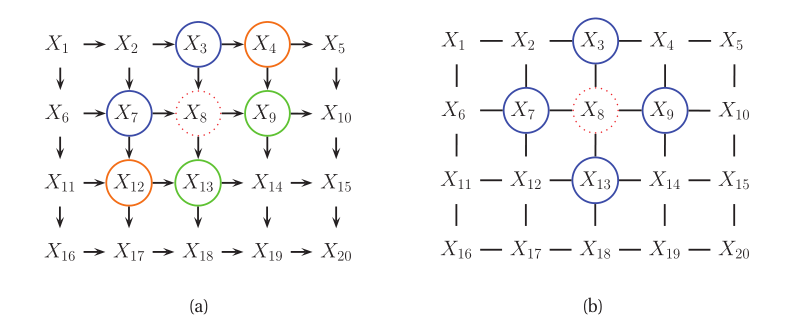1.2有向图和无向图得粗略对比

相比有向图，无向图得主要优点有是：无向图的边是对称的，这对某些领域，例如图像处理很有用
相比有向图，无向图的主要缺点是：
①这些参数的可解释性较差，模块化程度也较低；
②参数估计的计算成本更高

2. 无向图的一些条件独立性质
2.1 全局马尔可夫性质(关键性质)

对于节点集合A,B,C，当且仅当C把A、B进行分离时，A⊥B|C.
这句话意思就是说：把集合C中所有节点都去掉之后，A,B之间没有任何连接。
这个性质被称为无向图的全局马尔科夫性质(Global Markov Property for UGMs)
举个栗子：

$\{ 1,2\} \bot \{ 6,7\} |\{ 3,4,5\}$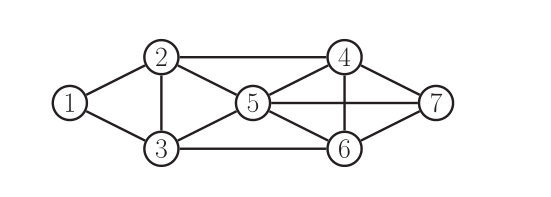2.2 无向图的局部马尔科夫性质

在无向图中，一个节点的马尔可夫毯子(Markov Blanket)就是该节点的直接邻居，根据Markov Blanket的定义，我们有：

$t \bot V\backslash cl(t)|mb(t)$
$cl(t) \triangleq mb(t) \cup \{ t\}$
2.3 成对马尔科夫性质

从局部马尔科夫性质我们可以推到出成对马尔科夫性质，他是这样定义的：
对于任意两个没有边连接的节点，给定剩下的节点，则这两个节点条件独立，即

$s \bot t|V\backslash \{ s,t\} \Leftrightarrow {G_{st}} = 0$
2.4 性质总结

很明显，全局马尔可夫(G)隐含局部马尔可夫，局部马尔可夫(L)隐含成对马尔可夫§。
可以证明，他们是等价的,即

$G \Leftrightarrow L \Leftrightarrow P$
3. 无向图模型的参数
3.1 无向图模型的一个弊端

尽管无向图模型的条件独立性质比(CI)有向图模型要简单的多，但是，在表示联合概率时，无向图就很逊色，这也是无向图的一个弊端。

3.2 解决方案
3.2.1 团与最大团的定义

无向图G中任何两个节点均有边连接的节点子集称为团(Clique)。若C是无向图G的一个团，并且不能再加进去任何一个G的节点使其成为一个更大的团，则称C为最大团(Maximual clique)

3.2.2 势函数(potential function)

我们在图G中的每一个最大团定义一个势函数，我们将最大团c的势函数表示为如下：

${\psi _c}({y_c}|{\theta _c})$

势函数可以为关于参数的任意非负函数。
从而我们将联合概率分布定义为：正比于所有团上势函数的乘积

3.2.3 Hammersley-Clifford定理

一个正的分布p(y) > 0 满足无向图G的条件独立性质当且仅当p可以被表示为因子的乘积，每一项因子为一个最大团上的势函数乘积，即

$p(y|\theta ) = \frac{1}{{Z(\theta )}}\prod\limits_{c \in C} {{\psi _c}({y_c}|{\theta _c})}$
其中，C是(最大)团的集合，Z（θ）是归一化因子也成为划分函数，定义如下：
$Z(\theta ) \triangleq \sum\limits_x {\prod\limits_{c \in C} {{\psi _c}({y_c}|{\theta _c})} }$
4. 无向图模型和统计物理学之间的联系

无向图模型和统计物理学有着很深的联系。具体地，有一个模型叫做吉布斯分布(Gibbs distribution)，它可以被写成如下形式：

$p(y|\theta ) = \frac{1}{{Z(\theta )}}\exp ( - \sum\limits_c {E({y_c}|{\theta _c})} )$
其中E(yc)>0,是关于团c中变量的一种能量，我们可以把这个模型转换为无向图模型(UGMs)通过定义势函数如下：
${\psi _c}({y_c}|{\theta _c}) = \exp ( - \sum\limits_c {E({y_c}|{\theta _c})} )$
我们看到高概率态对应于低能组态。这种模型经常被应用在物理学中(Ising Model)

有一点需要注意以下：我们可以自由地将参数化限制在图的边，而不是最大团，这个性质被称为逐对马尔可夫随机场(Pairwise MRFs)

$p(y|\theta ) \propto {\psi _{12}}({y_1},{y_2}){\psi _{13}}({y_1},{y_3}){\psi _{23}}({y_2},{y_3}){\psi _{24}}({y_2},{y_4})...{\psi _{35}}({y_3},{y_5})$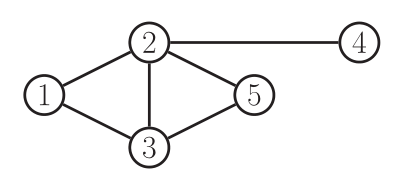这种形式由于它的简单性被被广泛的应用，但它不作为联合概率的一般形式。
参考文献
《Machine Learning A Probability Perspective》
《统计学方法，李航》


展开全文人工智能 机器学习
• 马尔科夫随机场（Markov Random Field，MRF）是典型的马尔科夫网，这是一种著名的无向图模型。图中每个节点表示一个或一组变量，节点之间的边表示两个变量之间的依赖关系。马尔科夫随机场有一组势函数，也成为“因子...
注：由于在CSDN中画图和输入公式非常麻烦，所以我选择了手写后拍照截图来表示，可能稍有模糊，请各位看官多担待。
下面开始正文叙述：
马尔科夫随机场（Markov Random Field，MRF）是典型的马尔科夫网，这是一种著名的无向图模型。图中每个节点表示一个或一组变量，节点之间的边表示两个变量之间的依赖关系。马尔科夫随机场有一组势函数，也成为“因子”，这是定义在变量子集上的非负实数函数，主要用于定义概率分布函数。
下图给出了一个典型的马尔科夫随机场：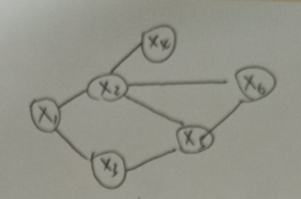图一
一、团和极大团
对于图中节点的一个子集，若其中任意两节点都有边连接，则称该节点子集为一个“团”。若在一个团中任意加入另外任何一个节点都不再形成“团”，则称该团委“极大团”。
例如，上图中{x1, x2} ,{x1, x3}, {x2,x4}, {x2， x5}, {x2, x6}, {x3, x6}, {x5, x6}, 和{x2,x5,x6}都是团，并且除了 {x2， x5}, {x2, x6}，{x5, x6}之外都是极大团。
显然，每个节点至少出现在一个极大团中。
二、联合概率分布
在马尔科夫随机场中，多个变量之间的联合概率分布能基于团分解为多个因子的乘积，每个因子仅与一个团相关。具体来说，对于n个变量x={x1,x2,…,xn}，所有团构成的集合为C，与团Q∈C对应的变量集合记为xQ ，则联合概率分布P(x)定义为：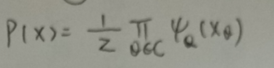其中，φQ 为与团Q对应的势函数，用于对团Q中的变量关系进行建模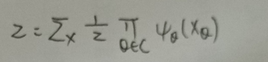Z为规范因子，以确保P(x)是被正确定义的概率。在实际应用中，往往很难精确计算Z非常困难，但许多任务往往不需要获得Z 的精确值。
显然，若变量个数较多，则团的数目将会很多（因为所有互相连接的两个变量都会构成团），这就意味着联合概率分布会有许多乘积项，显然会给计算带来负担。由于团 Q 不是极大团，因此它必被一个极大团 Q* 所包含，即 xQ ⊆ xQ* 。这就意味着变量 xQ 之间的关系不仅体现在势函数  φQ 中，还体现在 φQ* 中。
于是，联合概率分布P(x) 可以用极大团来定义：
假定所有极大团构成的集合为C* , 则有：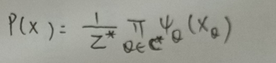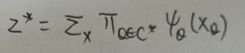由极大团的联合概率分布可知，图一可表示为：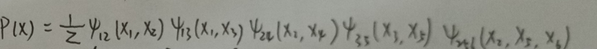其中，势函数 φ256(x2,x5,x6) 定义在极大团 {x2,x5,x6} ，由于它的存在，是的我们不需在为团 {x2， x5}, {x2, x6},{x5, x6}构建势函数。
三、条件独立性
在马尔科夫随机场中如何得到“条件独立性”？
同样，借助分离的概念。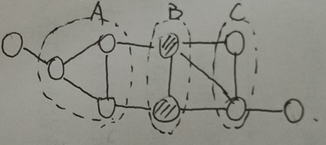如上图所示，若从节点集A中的节点B中的节点都必须经过节点机C中的节点，则称节点集A和B被节点集C分离，C称为“分离集”。对马尔科夫随机场而言，有：

“全局马尔科夫性”：给定两个变量子集的分离集，则这两个变量子集条件独立。

也就是说，上图中，若令A,B和C对应的变量集分别为 xA, xB和xC ，则xA 和xB 在给定的xC 的条件下独立，记为：证明条件独立性的成立：
为了便于证明，令上图的A,B,C分别对应单变量xA, xB和xC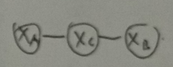图二
有联合概率分布：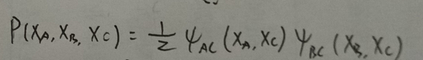由条件概率分布的定义可知：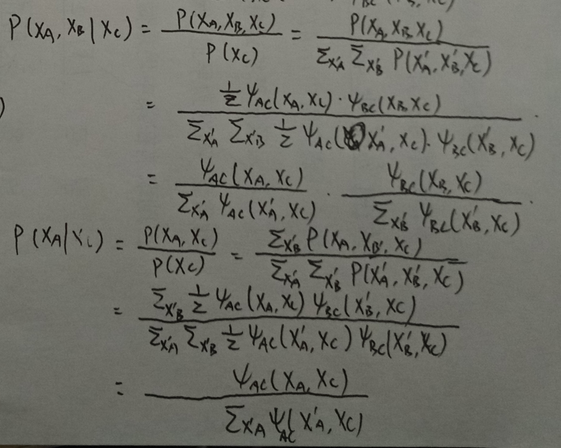因此有：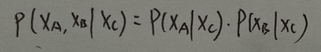即xA和xB在给定xC 时条件独立
四、推论
由全局马尔科夫性可得到两个很有用的推论：

局部马尔科夫性：给定某变量的邻接变量，则该变量条件独立于其他变量。形式化如下：
令 V 为图的节点集，n(v) 为节点 v 在图上的邻接节点， n*(v) = n(v)∪{v}， 有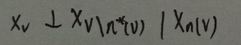成对马尔科夫性：给定所有其他变量，两个非邻接变量条件独立。形式化如下：
令图的节点集和边集分别为V和E，对图中的两个节点u和v，若<u,v>∈\E(边uv不在边集E中)，则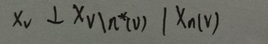五、势函数
现在考量势函数。显然，势函数ψQ(xQ) 的作用是定量刻画变量集xQ 中变量之间的相关关系。它应该是非负函数，且在所偏好的变量取值上有较大函数值。例如，图二中，假定变量变量均为二值变量，若势函数为：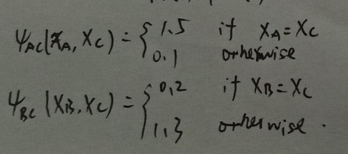说明该模型偏好变量xA 和 xC 拥有相同的值，xB和xC 有不同的取值。也就是xA 和 xC相同且xB和xC不停的变量值将取得较高的联合概率。
为了满足非负性，指数函数常被用于定义势函数，即：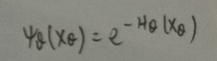HQ(xQ) 是一个定义在变量xQ 上的实值函数，常见形式为：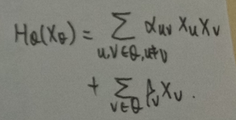其中αuv 和 βv 是参数。上式中的第二项仅考虑单节点，第一项则考虑每一对节点的关系。


展开全文机器学习 马尔科夫模型
• 马尔可夫网络和贝叶斯网络的联合概率分布是什么？...马尔可夫网络的联合概率分布是概率无向图模型，多个变量间的联合概率分布可以基于团分解维多个势函数的乘积，势函数只与一个团相关。 中文分...
• @概率图模型（推理：消息传递算法） 消息传递算法 链接: https://www.cnblogs.com/qiu-hua/p/13040954.html. 概率图模型G(V,E)由节点V和边E构成。在之前马尔科夫模型相关的博客中，我谈到马尔科夫模型的本质是当两个...算法
• 马尔科夫随机场MRF联合概率分解...MRF联合概率分解的另一个概念是potential function（势函数），联合概率分解成一系列potential函数的乘积。 Xc是最大团的所有结点。 一个potential function，是最大团的一个...人工智能 matlab
• 每个节点表示一个或一组变量，节点之间之间的边表示两个变量之间的依赖关系，马尔可夫随机场有一组势函数，定义在变量子集上的非负实函数，主要用于定义概率分布函数。 **对于中节点的一个子集，若其中任意两节点...
• 其利用特征函数以及参数的方式对势函数进行定义，可获得较好的效果。在之前有向的学习中，我们发现可以利用d-seperet，充分统计，狄利克雷函数等方式来很优雅的获得参数估计的解析解。但是在无向中，这些优越的...
• 概率图模型G(V,E)由节点V和边E构成。在之前马尔科夫模型相关的博客中，我谈到马尔科夫模型的本质是当两个人交流后，其意见（两个随机变量）同意0与不同意1的概率组合。而势函数表达的是两个意见相同或者相左的程度。...
• 实际上，因子是整个概率图的核心。对于有向图而言，因子对应的是CPD（条件分布）；对无向图而言，因子对应的是势函数。总而言之，因子是一个映射，将随机变量空间映射到实数空间。因子表现的是对变量之间关系的一种...
• 节点势函数初始化； 所有消息初始化为 1； 选取所有边，迭代更新消息 ； 当消息传递收敛时，计算所有节点的信念（belief） 三、BP 算法与Bethe 聚类 四、BP 算法与团树传播算法的联系 ...
• Koller 教授把决策作为一种单独的模块进行讲解，但我认为，决策和推理本质上是一样的，都是在假设已知CPD或者势函数的情况下对模型给出结论。 1、决策==逐利 　决策的基本思想很intuitive，并且非常有用。在赌博...
• 首先为样本数据构建概率无向图模型,利用极大团和势函数计算无向图中数据样本的概率密度,将此概率密度作为一种聚类先验知识注入近邻传播算法的偏向参数中,提高算法的聚类效率;并用高斯降噪和簇归并方法进一步提升算法...
• 概率无向图 定义 概率无向图模型（Probabilistic Undirected Graphical Model）是一个可以用无向图表示的联合概率分布。 它的整体结构是一张图（Graph），图中每一个节点...势函数和团 关于马尔可夫随机场，有几个...人工智能 机器学习
• 马尔可夫随机场有一组势函数（potential functions），亦称“因子”（factor），这是定义在变量子集上的非负实函数，主要用于定义概率分布函数。 2显示出一个简单的马尔可夫随机场。对于中节点的
• 为了快速有效地从遥感图像中提取目标建筑物...选择多幅遥感图片进行实验，结果表明,采用分解层数为3的Haar小波，类别数为2，MLL模型势函数β为1时，该方法能够完成复杂背景下的建筑物目标分割并能对规则目标进行检测。
• 流 这是论文的官方资料库 黄进伟，Ricky TQ Chen，Christos Tsirigotis和Aaron Courville撰写的“凸潜在流：具有最佳传输和凸优化的通用概率分布”。 在ICLR 2021中。[ ] [ ] 依存关系： 运行pip install -r ...Python
• 上篇主要介绍了概率图模型，首先从生成式模型与判别式模型的定义出发，引出了概率图模型的基本概念，即利用图结构来表达变量之间的依赖关系；接着分别介绍了隐马尔可夫模型、马尔可夫随机场、条件随机场、精确推断...
• 上篇主要介绍了概率图模型，首先从生成式模型与判别式模型的定义出发，引出了概率图模型的基本概念，即利用图结构来表达变量之间的依赖关系；接着分别介绍了隐马尔可夫模型、马尔可夫随机场、条件随机场、精确推断...
• 上篇主要介绍了概率图模型，首先从生成式模型与判别式模型的定义出发，引出了概率图模型的基本概念，即利用图结构来表达变量之间的依赖关系；接着分别介绍了隐马尔可夫模型、马尔可夫随机场、条件随机场、精确推断...
• 参考：概率无向图模型 1. 概率无向图的因子分解 注：有一点笔者仍然不明白，《统计学习方法》是定义P(Y)的乘积是在最大团上进行，而有材料说明乘积是在极大团上进行。 无向图中的极大团的结点个数可以是不同的，...条件随机场
• 基于概率图模型定义的联合概率分布，我们能对目标变量的边际分布或以某些可观测变量为条件的条件分布进行推断。边际分布是指对无关变量求和或积分后得到的结果，例如在马尔可夫网中，变量的联合分布呗表示成极大团的...周志华 机器学习